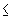## Project 18: Spanning Tree Versus Graph Distance

If G is a connected graph (no loops or multiple edges) then G has a spanning tree T. (A spanning tree in a graph is a connected subgraph which includes all the vertices of the graph and is a tree.)

If u and v are two vertices in G then one can compare the distance between u and v in G and the distance between u and v using only edges of the spanning tree T. We will denote the two distances involved by dG (u,v) and dT (u,v). The distances between two vertices in the tree is at least as large as the distance between the vertices in the original graph. When the difference between dG (u,v) and dT (u,v) isk for every pair of vertices u and v in G, then T is called a k-approximating tree for k.

Problems

1. Study the relationship between the diameter d of G, and the extent to which dT (u,v) can be used to approximate dG (u,v) closely (i.e. the value of k within which T approximates G)?

2. Compare how well a spanning tree can be used to approximate distances in G when G has a unique center, compared with the case when every vertex of G is in the center.

3. Does a spanning tree in a graph which mininimizes the average "discrepancy" between tree and graph distances (over the set of all spanning trees) have any nice properties?

Joseph Malkevitch
Department of Mathematics and Computing
York College (CUNY)
Jamaica, New York 11451-0001
email: malkevitch@york.cuny.edu
(Comments and results related to the project above are welcome.)

Acknowledgements
Some of this work was prepared with partial support from the National Science Foundation (Grant Number: DUE 9555401) to the Long Island Consortium for Interconnected Learning (administered by SUNY at Stony Brook, Alan Tucker, Project Director).